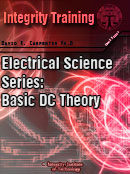### Basic DC Theory - 4 PDH's/\$55.00 (Module 2 from Fundamentals of Electrical Science Series - PDF format)Basic DC Theory - PDFFull description:Topics covered in this module: DC Sources: Batteries, DC Generator, Thermocouples, Rectifiers, Forward Bias, Reverse Bias, Half-Wave Rectifier Circuit, Full-Wave Rectifier Circuit, and SummaryDC Circuit Terminology: Schematic Diagram, One-Line Diagram, Block Diagram, Wiring Diagram, Resistivity, Temperature Coefficient of Resistance, Electric Circuit, Series Circuit, Parallel Circuit, Equivalent Resistance, and SummaryBasic DC Circuit Calculations: Series Resistance, Parallel Currents, Resistance in Parallel, Simplified Formulas, Voltage Divider, Current Division, and SummaryVoltage Polarity and Current Direction: Conventional and Electron Flow, Polarities and SummaryKirchhoffs Law: Kirchhoffs Laws, Kirchhoffs Voltage Law, Applying Kirchhoffs Current Law, and SummaryDC Circuit Analysis: Loop Equations, Node Equations, Series-Parallel Circuit Analysis, Y and Delta Network Calculation, and SummaryDC Circuit Faults: Open Circuit (Series), Open Circuit (Parallel), Short Circuit (Series), Short Circuit (Parallel), and Summary